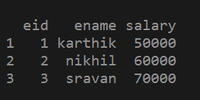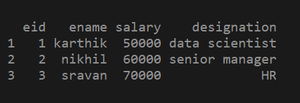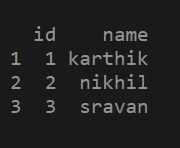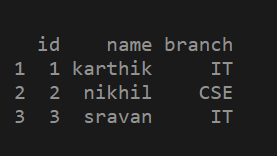How to add column to dataframe in R ?

• Last Updated : 26 Mar, 2021

In this article, we are going to see how to add columns to dataframe in R. First, let’s create a sample dataframe.

We can add a column to a data frame using \$ symbol.

syntax: dataframe_name \$ column_name = c( value 1,value 2 . . . , value n)

Here c() function is a vector holds values .we can pass any type of data with similar type.

Steps for adding a column to a dataframe.

1. Create a data frame.
2. Use the \$ symbol as shown in the above syntax to add a column to a data frame.
3. Print the updated data frame to see the changes.

Example 1: Creating a data frame.

R

 # Creating a data frame with eid , ename,salary as columnsdf2 = data.frame(eid = c(1, 2, 3),                 ename = c("karthik", "nikhil", "sravan"),                 salary = c(50000, 60000, 70000))  # printing the data frameprint(df2)Adding a column using \$ symbol:

R

 # creating a data framedf2 = data.frame(eid = c(1, 2, 3),                 ename = c("karthik", "nikhil", "sravan"),                 salary = c(50000, 60000, 70000))  # adding a new column to the data frame using \$ symboldf2\$designation = c("data scientist", "senior manager", "HR")   # printing the updated data frameprint(df2)Example 2: Creating a data frame

R

 # creating a data frame using vectorsdf1 = data.frame(id = c(1, 2 ,3),                 name = c("karthik", "nikhil", "sravan"))  # printing the data frameprint(df1)Adding a column to the data frame

R

 # creating a data framedf1 = data.frame(id = c(1, 2, 3),                 name = c("karthik", "nikhil", "sravan"))# adding a new column to the data frame using \$ symboldf1\$branch = c("IT", "CSE", "IT")  # printing the updated data frameprint(df1)My Personal Notes arrow_drop_up